# FIR Halfband Interpolator

Interpolate signal using polyphase FIR half band filter

•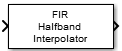Libraries:
DSP System Toolbox / Filtering / Multirate Filters

## Description

The FIR Halfband Interpolator block performs interpolation of the input signal by a factor of two. The block uses an FIR equiripple design or a Kaiser window design to construct the halfband filters. The block uses an efficient polyphase implementation to filter the input. The implementation takes advantage of the zero-valued coefficients of the FIR halfband filter, making one of the polyphase branches a delay. For more information, see Algorithms. You can also use this block to implement the synthesis portion of a two-band filter bank to synthesize a signal from lowpass and highpass subbands.

The input signal can be a real- or complex-valued column vector or a matrix. If the input signal is a matrix, the block treats each column of the matrix as an independent channel. The block supports fixed-point operations and ARM® Cortex® code generation. For more information on ARM Cortex code generation, see Code Generation for ARM Cortex-M and ARM Cortex-A Processors.

The block also supports SIMD code generation. For details, see Code Generation.

## Examples

expand all

Design and implement an FIR halfband interpolator using the FIR Halfband Interpolator block. Pass a noisy input through the interpolator. Plot the spectrum of the input and the interpolated output in the spectrum analyzer.

Open and inspect the model. The input is a noisy sinusoidal signal with two frequencies, one at 1 kHz and the other at 15 kHz. Add white Gaussian noise with a mean of 0 and a variance of 0.05 to this signal.

The FIR halfband interpolator has a transition width of 4.1 kHz and a stopband attenuation of 80 dB. Visualize the magnitude response of the filter by clicking the View Filter Response button in the block dialog box.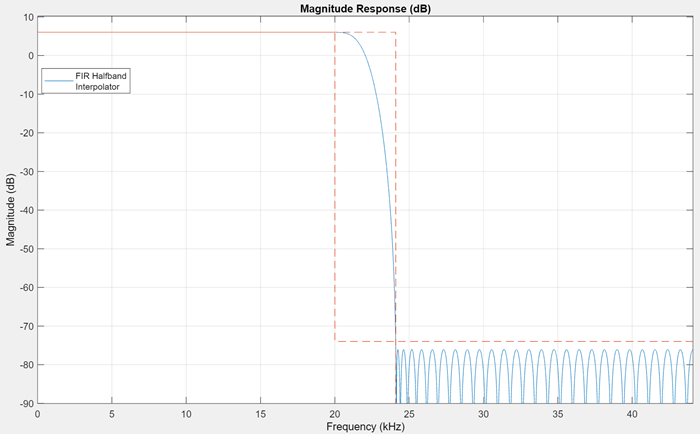Pass the noisy sinusoidal signal through the interpolator. Plot the spectrum of the input and the interpolated output in the spectrum analyzer.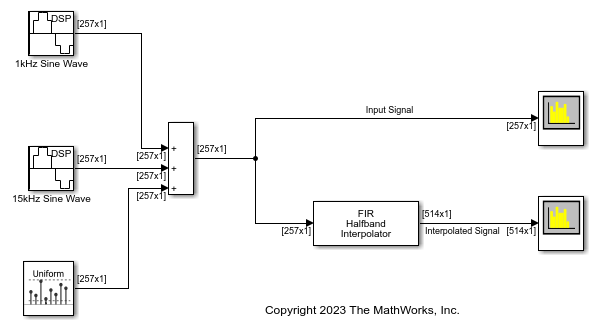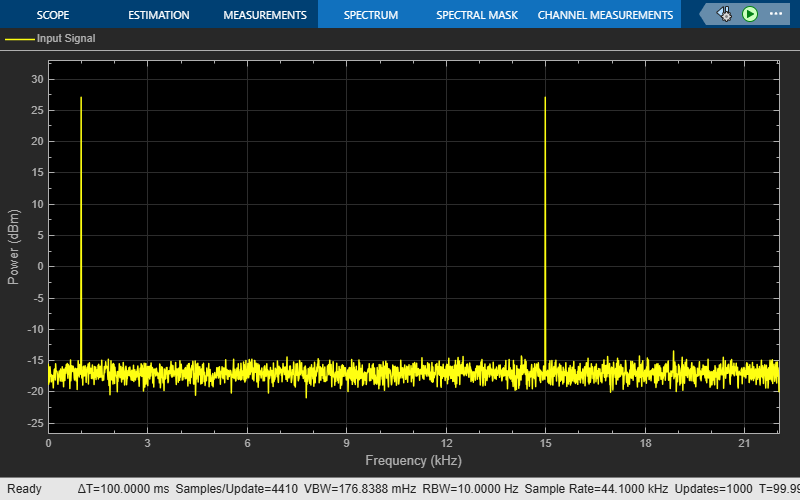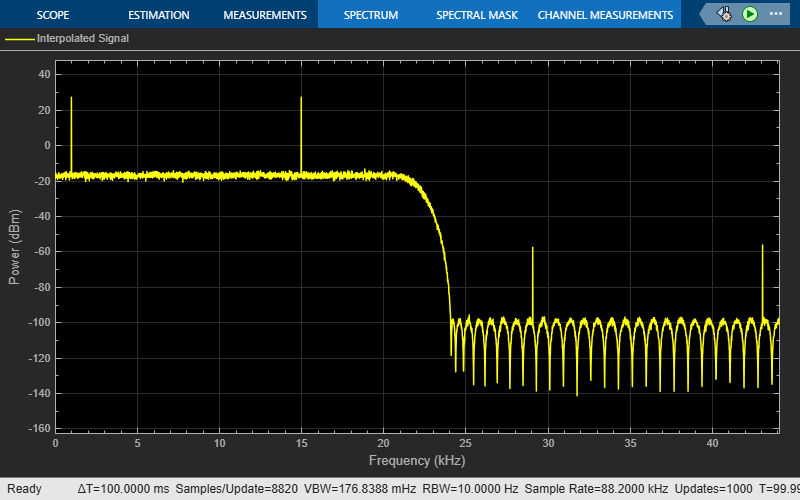Since R2023b

Use the FIR Halfband Decimator and FIR Halfband Interpolator blocks to extract and reconstruct the low-frequency subband from a speech signal.

Open and inspect the model. The input audio data is a single-channel speech signal with the sample rate of 22050 Hz.

Specify the Sample rate mode parameter of the FIR Halfband Decimator and FIR Halfband Interpolator blocks to `Use normalized frequency (0 to 1)`. This option enables you to specify the transition width of the decimation and interpolation filters in normalized frequency units. Set the transition width to 0.093 in normalized frequency units and the stopband attenuation to 80 dB. The design method is set to `Auto` by default. In the `Auto` mode, the block selects the equiripple or Kaiser window design method based on the design parameters of the filter.

Read the speech signal from the audio file in frames of 1024 samples. The FIR Halfband Decimator block extracts and outputs the lowpass subband of the speech signal. The FIR Halfband Interpolator block reconstructs the lowpass approximation of the speech signal by interpolating the lowpass subband.

The Audio Device Writer block plays the filtered output.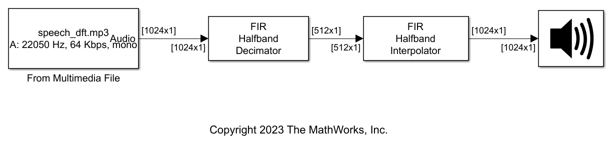Use the FIR Halfband Decimator and FIR Halfband Interpolator blocks to implement a two-channel filter bank. This example uses an audio file input and shows that the power spectrum of the filter bank output does not differ significantly from the input. Play the output of the filter bank using the Audio Device Writer block.

Open and inspect the model. The input audio data is a single-channel speech signal with a sample rate of 22050 Hz.

The FIR Halfband Decimator block acts as an FIR halfband analysis bank as the Output highpass subband parameter is selected in the block dialog box. The FIR Halfband Interpolator block acts as an FIR halfband synthesis bank as the Input highpass subband parameter is selected in the block dialog box.

Set the Sample rate mode parameter in the FIR Halfband Decimator and FIR Halfband Interpolator blocks to `Inherit from input port` so that the blocks inherit the sample rate from the respective input ports. Set the transition width to 4.1 kHz and the stopband attenuation to 80 dB. The design method is set to `Auto` by default. In the `Auto` mode, the block selects the equiripple or Kaiser window design methods based on the design parameters of the filter.

Read the speech signal from the audio file in frames of 1024 samples. The FIR halfband analysis bank extracts the lowpass and highpass subbands of the speech signal. The FIR halfband synthesis filter bank synthesizes the speech signal from the lowpass and highpass subbands.

Display the power spectrum of the audio input and the output of the synthesis filter bank in the spectrum analyzer. Play the synthesized speech signal using the Audio Device Writer block.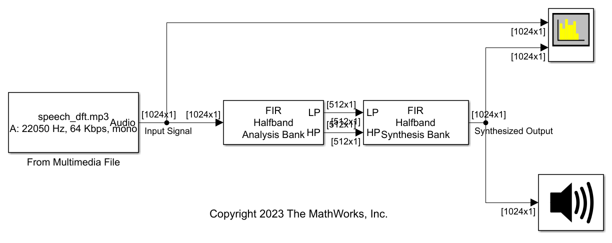## Ports

### Input

expand all

Specify the data input as a vector or a matrix. If the input signal is a matrix, the block treats each column of the matrix as an independent channel.

When you select the Input highpass subband parameter, this block acts as a halfband synthesis filter bank. The input at this port is then the lowpass subband output of a halfband analysis filter bank.

This port is unnamed until you select the Input highpass subband parameter.

Data Types: `single` | `double` | `int8` | `int16` | `int32` | `int64` | `uint8` | `uint16` | `uint32` | `uint64` | `fixed point`
Complex Number Support: Yes

Specify the second input to the synthesis filter bank as a column vector or a matrix. This signal is the highpass subband output of a halfband analysis filter bank. If the input signal is a matrix, the block treats each column of the matrix as an independent channel.

The size, data type, and complexity of both the inputs must be the same.

#### Dependency

To enable this port, select the Input highpass subband parameter.

Data Types: `single` | `double` | `int8` | `int16` | `int32` | `int64` | `fixed point`
Complex Number Support: Yes

### Output

expand all

Output of the FIR halfband interpolator, returned as a column vector or a matrix. The number of rows in the interpolator output is twice the number of rows in the input signal.

When the output is fixed-point, it is signed only.

Data Types: `single` | `double` | `int8` | `int16` | `int32` | `int64` | `fixed point`
Complex Number Support: Yes

## Parameters

expand all

### Main Tab

Select the parameters that the block uses to design the FIR halfband filter.

• ```Transition width and stopband attenuation``` (default) — Design the filter using Transition width (Hz) and Stopband attenuation (dB). This design is the minimum order design.

• ```Filter order and transition width``` — Design the filter using Filter order and Transition width (Hz).

• ```Filter order and stopband attenuation``` — Design the filter using Filter order and Stopband attenuation (dB).

• `Coefficients` — Specify the filter coefficients directly through the Numerator parameter.

Specify the transition width as a real positive scalar in Hz or in normalized frequency units (since R2023b).

If you set the Sample rate mode parameter to:

• `Specify on dialog` or `Inherit from input port` –– The value of the transition width is in Hz and must be less than half the value of the output sample rate (2 × input sample rate).

• ```Use normalized frequency (0 to 1)``` –– The value of the transition width is in normalized frequency units. The value must be a positive scalar less than `1.0`.

(since R2023b)

#### Dependencies

To enable this parameter, set Filter specification to ```Filter order and transition width``` or ```Transition width and stopband attenuation```.

Specify the filter order as an even positive integer.

#### Dependencies

To enable this parameter, set Filter specification to ```Filter order and transition width``` or ```Filter order and stopband attenuation```.

Specify the stopband attenuation as a real positive scalar in dB.

#### Dependencies

To enable this parameter, set Filter specification to ```Filter order and stopband attenuation``` or ```Transition width and stopband attenuation```.

Specify the FIR halfband filter coefficients directly as a row vector. The coefficients must comply with the FIR halfband impulse response format. If (`length`(`Numerator`) − 1)/2 is even, where (`length`(`Numerator`) − 1) is the filter order, every other coefficient starting with the first coefficient must be 0 except the center coefficient which must be 1.0. If (`length`(`Numerator`) − 1)/2 is odd, the sequence of alternating zeros with 1.0 at the center starts at the second coefficient.

#### Dependencies

To enable this parameter, set Filter specification to `Coefficients`.

Specify the filter design method as one of the following:

• `Auto` –– The algorithm automatically chooses the filter design method depending on the filter design parameters. The algorithm uses the equiripple or the Kaiser window method to design the filter.

If the design constraints are very tight, such as very high stopband attenuation or very narrow transition width, then the algorithm automatically chooses the Kaiser method, as this method is optimal for designing filters with very tight specifications. However, if the design constraints are not tight, then the algorithm chooses the equiripple method.

When you set the Design method parameter to `Auto`, you can determine the method used by the algorithm by examining the passband and stopband ripple characteristics of the designed filter. If the block used the equiripple method, the passband and stopband ripples of the designed filter have a constant amplitude in the frequency response. If the filter design method the block chooses in the `Auto` mode is not suitable for your application, manually specify the Design method as `Equiripple` or `Kaiser`.

• `Equiripple` –– The algorithm uses the equiripple method.

• `Kaiser` –– The algorithm uses the Kaiser window method.

#### Dependencies

To enable this parameter, set Filter specification to ```Filter order and stopband attenuation```, ```Filter order and transition width```, or ```Transition width and stopband attenuation```.

When you select this check box, the block acts as a synthesis filter bank. The block accepts two inputs to synthesize: lowpass and highpass subbands. When you clear this check box, the block acts as an IIR half band interpolator and accepts a single vector or matrix as input. By default, this check box is cleared.

Since R2023b

Specify the input sample rate using one of these options:

• `Specify on dialog` –– Specify the input sample rate in the block dialog box using the Input sample rate (Hz) parameter.

• `Inherit from input port` –– The block inherits the sample rate from the input signal.

• ```Use normalized frequency (0 to 1)``` –– Specify the passband-edge and the stopband-edge frequencies in normalized frequency units (0 to 1).

#### Dependencies

To enable this parameter, set Filter specification to any value other than `Coefficients`.

Specify the sample rate of the input signal as a scalar in Hz.

#### Dependencies

To enable this parameter, set:

• Filter specification to any value other than `Coefficients`.

• Sample rate mode to `Specify on dialog`.

(since R2023b)

Click this button to open the Filter Visualization Tool (FVTool) and display the magnitude and phase response of the FIR Halfband Interpolator. The response is based on the values you specify in the block parameters dialog box. Changes made to these parameters update FVTool.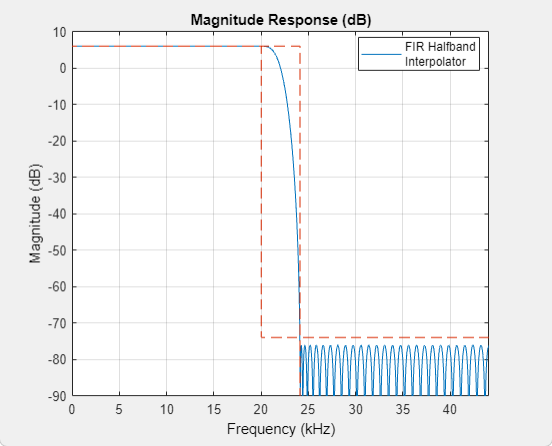To update the magnitude response while FVTool is running, modify the dialog box parameters and click .

Specify the type of simulation to run. You can set this parameter to:

• `Code generation` (default)

Simulate model using generated C code. The first time you run a simulation, Simulink® generates C code for the block. The C code is reused for subsequent simulations, as long as the model does not change. This option requires additional startup time but provides faster simulation speed than ```Interpreted execution```.

• `Interpreted execution`

Simulate model using the MATLAB®  interpreter. This option shortens startup time but has slower simulation speed than ```Code generation```.

### Data Types Tab

Specify the rounding method for the output fixed-point operations. The rounding methods are `Ceiling`, `Convergent`, `Floor`, `Nearest`, `Round`, `Simplest`, and `Zero`. The default is `Floor`.

Specify the fixed-point data type of the coefficients as one of the following:

• `fixdt(1,16)` (default) — Signed fixed-point data type of word length `16` with binary point scaling. The block determines the fraction length automatically from the coefficient values in such a way that the coefficients occupy maximum representable range without overflowing.

• `fixdt(1,16,0)` — Signed fixed-point data type of word length `16` and fraction length, `0`. You can change the fraction length to any other integer value.

• `<data type expression>` — Specify the coefficients data type by using an expression that evaluates to a data type object. For example, `numerictype`(`fixdt`(```[ ]```,`18`, `15`)). Specify the sign mode of this data type as `[ ]` or true.

• `Refresh Data Type` — Refresh to the default data type.

Click the buttonto display the data type assistant, which helps you set the coefficients data type.

## Block Characteristics

 Data Types `double` | `fixed point` | `single` Direct Feedthrough `no` Multidimensional Signals `no` Variable-Size Signals `yes` Zero-Crossing Detection `no`

expand all

expand all

## Version History

Introduced in R2015b

expand all